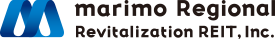1. Home
2. Portfolio
3. Portfolio Data

Portfolio Data

Portfolio Summary

As of January 4, 2019

• Total acquisition price
25,225million yen
• Number of properties
26properties
• Total appraisal value
29,207million yen
• Appraisal NOI yield
6.7%
• Average occupancy rate
98.3%
(Note 1) “Average occupancy rate” is the occupancy rate (ratio of total leased area to total leasable area of total leasable area) as of May 31, 2019, rounded to the first decimal place. “Appraisal NOI yield” is the figure calculated by dividing appraisal NOI as of December 31, 2018 by acquisition price, rounded to the first decimal place.

Portfolio Diversification

As of January 4, 2019

Investment ratio by building age

(Note 1) “Investment ratio by building age” is the figure as of December 31, 2018 rounded to the first decimal place.

PAGE TOP